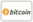Bookcover of Tensorial Invariants of Electrical Circuits
Booktitle:

# Tensorial Invariants of Electrical Circuits

LAP LAMBERT Academic Publishing (2013-09-24 )eligible for voucher
ISBN-13:

### 978-3-659-45942-9

ISBN-10:
3659459429
EAN:
9783659459429
Book language:
English
Blurb/Shorttext:
It is proved that to any electric circuit there correspond two pairs of of conjugate linear vector spaces. One of these pairs is generated by a homological group, while the other by a cohomological one. A new method of analysis of mechanical and electric circuits is proposed, which consists in representing the main variables and matrices of oscillatory circuits in terms of many-dimensional tensor objects. A solution is obtained for the problem of defining eigenvalues of pure-loop and pure-node circuits. It is shown that the latter circuits are pairwise equal to impedances' values of individual branches (to eigenvalues of a primitive circuit). A simple technique of separating roots of characteristic polynomials of many-dimensional systems with concentrated parameters is found. New matrix relations are obtained for loop and node matrices which make it possible to reduce the calculation of determinants of these matrices to the calculation of determinants of matrices of lower orders. A new method is developed for defining a full range of eigenvalues of linear oscillatory systems with a great number of degrees of freedom.
Publishing house:
Website:
https://www.lap-publishing.com/
By (author) :
Alexander Milnikov
Number of pages:
144
Published on:
2013-09-24
Stock:
Available
Category:
Electricity, magnetism, optics
Price:
44.90 €
Keywords:
electrical circuits, Linear Transformations, Eigen values, Homological and Cohomological Groups, Tensorial Invariants, Intermediate Problems### Categories